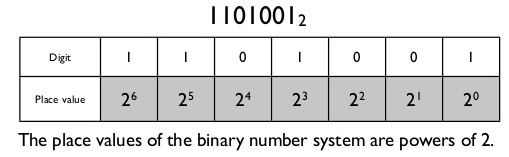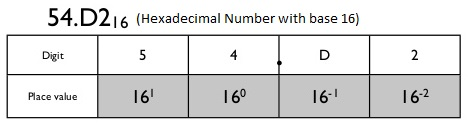# Hex to Decimal Conversion Simplified

Converting a hex to decimal is not difficult if you thoroughly understand what is mentioned on this page. The process of converting any hexadecimal number to its decimal or binary equivalent is a tricky one. If you are able to establish some rules you are lucky. But this is only possible if you see the things systematically. Also you will never need to use hexadecimal converter because you will get the conversion in no time.

The process of converting hex to decimal is same like we convert binary to decimal. The difference is the base of the numbering system i.e. 16 in case of hexadecimal numbers. You can find many apps and online hex converters which help you convert the hexadecimal numbers. But unfortunately we can not use them while inside the examination rooms or in class rooms.

### Place Value of a Digit

To convert any number to other base we must understand the place value. The place value for a binary digit is powers of 2, and power value starts with zero from right most digit and increases to left i.e. as mentioned below. Same is applicable for hexadecimal numbers.Also for a number with fractions the place value for digits on right side of point decreases with power of -1 to so on. Like below:## Omission of Zero Digit Values

After you find out the place values of each digit you have to multiply each digit with its place value. Finally sum all the obtained values to get the converted number in required base. In this way all place values corresponding to zero digits shall not exist.

Below is example of all steps involved for hex converting to decimal from 54.D2 using manual hexadecimal converter.#### hex to decimal conversion video – hex converter

Below is a very simple one minute video guide on how to convert hexadecimal numbers to decimal numbers. hexadecimal converter.

error: Content is protected !!